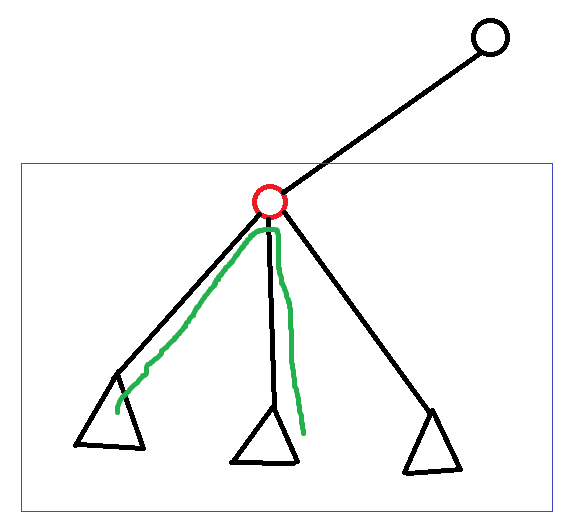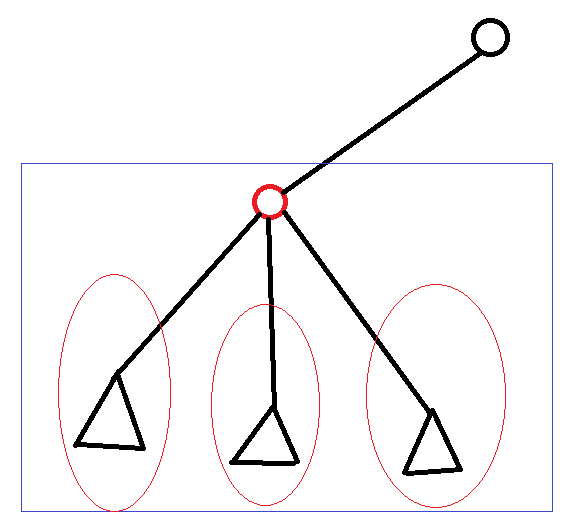# POJ 1741 Tree, 树的重心, 树分治, 点分治

POJ这一题应该算是树分治的入门题，顺便用这一题来详细说明树分治的一些具体内容。

http://poj.org/problem?id=1741

Tree
Time Limit: 1000MS Memory Limit: 30000K

Description

Give a tree with n vertices,each edge has a length(positive integer less than 1001).
Define dist(u,v)=The min distance between node u and v.
Give an integer k,for every pair (u,v) of vertices is called valid if and only if dist(u,v) not exceed k.
Write a program that will count how many pairs which are valid for a given tree.

Input

The input contains several test cases. The first line of each test case contains two integers n, k. (n<=10000) The following n-1 lines each contains three integers u,v,l, which means there is an edge between node u and v of length l.
The last test case is followed by two zeros.

Output

For each test case output the answer on a single line.

Sample Input

5 4
1 2 3
1 3 1
1 4 2
3 5 1
0 0


Sample Output

8

1.转化问题：我们将这棵树转化为一棵有根树，这样就可以统计 经过一个根节点 且只经过其子树中的节点的 合法路径 的条数，将经过各个点的这样的路径数加起来既是答案；如图，绿色的路径就是一条我们要求的路径；

2.如何得出经过每一个根结点的合法路径数呢？对于每一棵子树（如图中方框中 以红色结点为根的子树），我们dfs求出这棵子树中所有节点到红色根节点的距离,然后将这些距离放在dep数组中sort一下，这样就可以显然地求出这棵子树中所有合法路径条数；3.但这样求出的不是经过一个节点的合法路径数，而是整个子树中的合法路径数；这些路径中，有些路径并不经过根节点；显然，如果这样计算求和，会有很多路径被重复计算。为了去除重复，我们对红色根节点的所有儿子节点做和第2步相同的操作，并且将每个儿子的结果都剪掉；这样就剪掉了所有只经过子树中的结点、而不经过根节点的路径数。减去之后，剩下的自然就是我们要求的经过根节点的路径数。#include<cstdio>
#include<iostream>
#include<cstring>
#include<algorithm>
#include<vector>
using namespace std;
const int inf=1e5+10;

int maxn[inf],siz[inf],G,subsiz;
bool vis[inf];
int dp[inf<<1],dep[inf<<1];//dp[]存储到根节点的距离；dep[]是用来sort的，dep表示dep数组中元素的个数
int n,k,ans=0;

void init(void){
memset(vis,false,sizeof vis);
cnt=0;ans=0;
}

to[++cnt]=v;len[cnt]=w;
}

void getG(int u,int f){//找重心
siz[u]=1;maxn[u]=0;
int v=to[i];if (v!=f && !vis[v]){
getG(v,u);
siz[u]+=siz[v];
maxn[u]=max(maxn[u],siz[u]);
}

}maxn[u]=max(maxn[u],subsiz-siz[u]);
G=(maxn[u]<maxn[G])?u:G;
}

void dfs(int u,int f){//dfs确定每个点到根节点的距离
dep[++dep]=dp[u];
int v=to[i];if (v!=f && !vis[v]){
dp[v]=dp[u]+len[i];
dfs(v,u);
}
}
}

int calc(int u,int inidep){//inidep是这一点相对于根节点的初始距离
dep=0;
dp[u]=inidep;
dfs(u,0);
sort(dep+1,dep+1+dep);
int sum=0;
for (int l=1,r=dep;l<r;){//计算合法点对数目
if (dep[l]+dep[r]<=k) {sum+=r-l;l++;}
else r--;
}
return sum;
}

void divide(int g){	//递归，找到重心并以重心为根节点进行计算，再对子树递归处理
ans+=calc(g,0);
vis[g]=true;
int v=to[i]; if (!vis[v]){
ans-=calc(v,len[i]);
maxn=subsiz=siz[v];G=0;getG(v,0);
divide(G);
}
}
}

int main(){
while(scanf("%d%d",&n,&k)==2){
if (!n && !k) break;
init();
for (int i=1,u,v,w;i<n;i++){
scanf("%d%d%d",&u,&v,&w);
}
subsiz=maxn=n;G=0;getG(1,0);
divide(G);
printf("%d\n",ans);
}
return 0;
}

09-122938

#### [codevs 3639] 树的中心---树形DP(树的重心)

09-04587

#### POJ 1741:点分治详解10-31514

#### 【专题训练】医院设置 | 树的边、点都带权重心

06-0194

#### POJ 1741（点分治）（模板）

08-182103

#### 树上点分治详解【入门向】

05-03985

#### 专题·求树的重心，树的直径【including 例题扫雪系列 I、II

04-09954

#### POJ-1741 (点分治模板)

10-12203

#### poj 1741

06-0266

#### hdu 5015 233 Matrix（矩阵快速幂）

07-14487

#### 求树的重心©️2020 CSDN 皮肤主题: 大白 设计师: CSDN官方博客点击重新获取扫码支付1.余额是钱包充值的虚拟货币，按照1:1的比例进行支付金额的抵扣。
2.余额无法直接购买下载，可以购买VIP、C币套餐、付费专栏及课程。余额充值